## 数据结构与算法六：队列

### 理解“队列”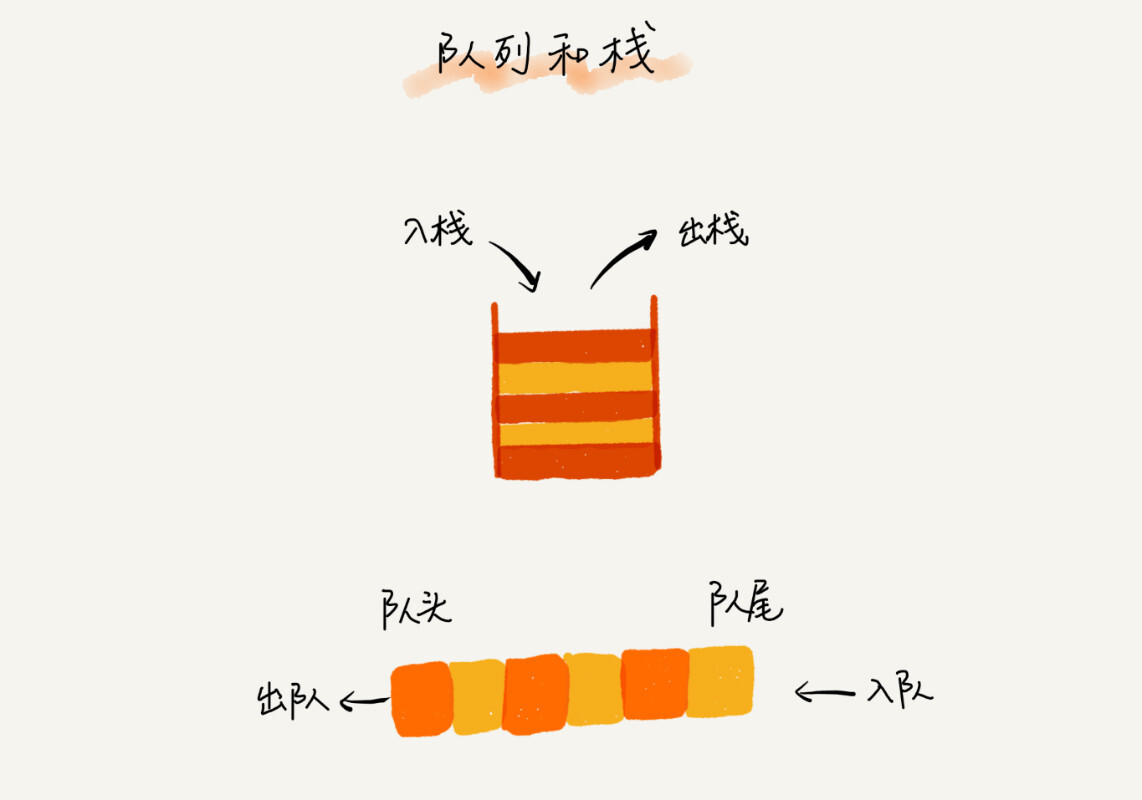### 顺序队列和链式队列

``````// 用数组实现的队列
public class ArrayQueue {
// 数组：items，数组大小：n
private String[] items;
private int n = 0;
private int tail = 0;

// 申请一个大小为capacity的数组
public ArrayQueue(int capacity) {
items = new String[capacity];
n = capacity;
}

// 入队
public boolean enqueue(String item) {
// 如果tail == n 表示队列已经满了
if (tail == n) return false;
items[tail] = item;
++tail;
return true;
}

// 出队
public String dequeue() {
if (head == tail) return null;
// 为了让其他语言的同学看的更加明确，把--操作放到单独一行来写了
return ret;
}
}``````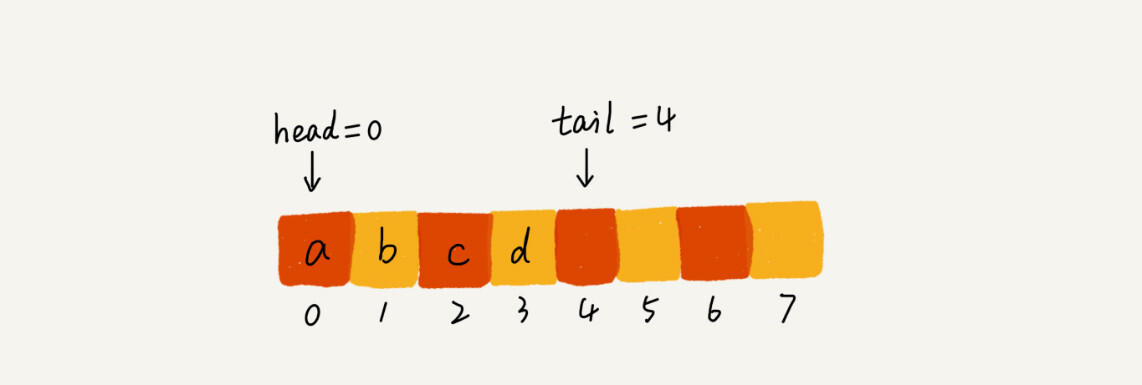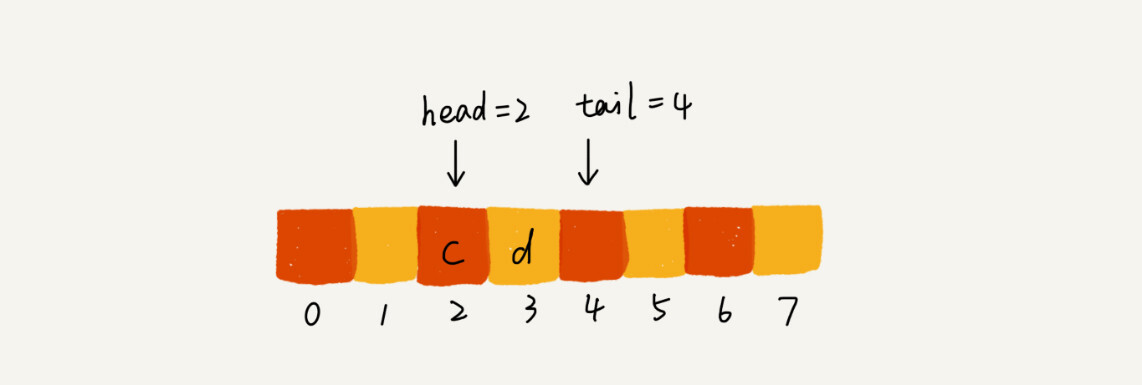``````   // 入队操作，将item放入队尾
public boolean enqueue(String item) {
// tail == n表示队列末尾没有空间了
if (tail == n) {
if (head == 0) return false;
// 数据搬移
for (int i = head; i < tail; ++i) {
}
}

items[tail] = item;
++tail;
return true;
}``````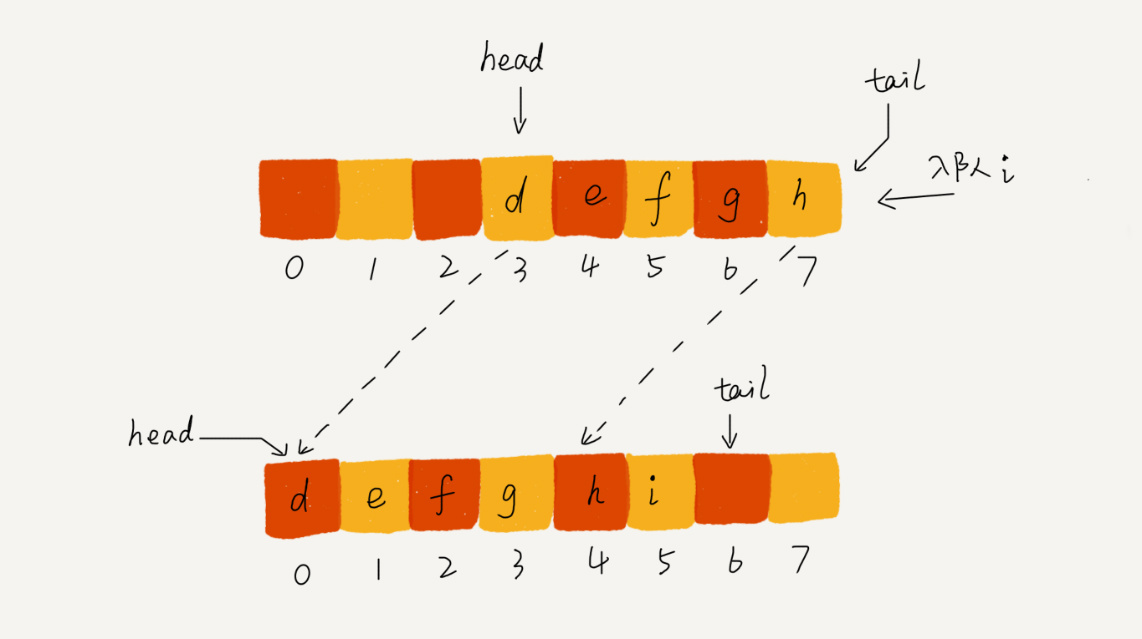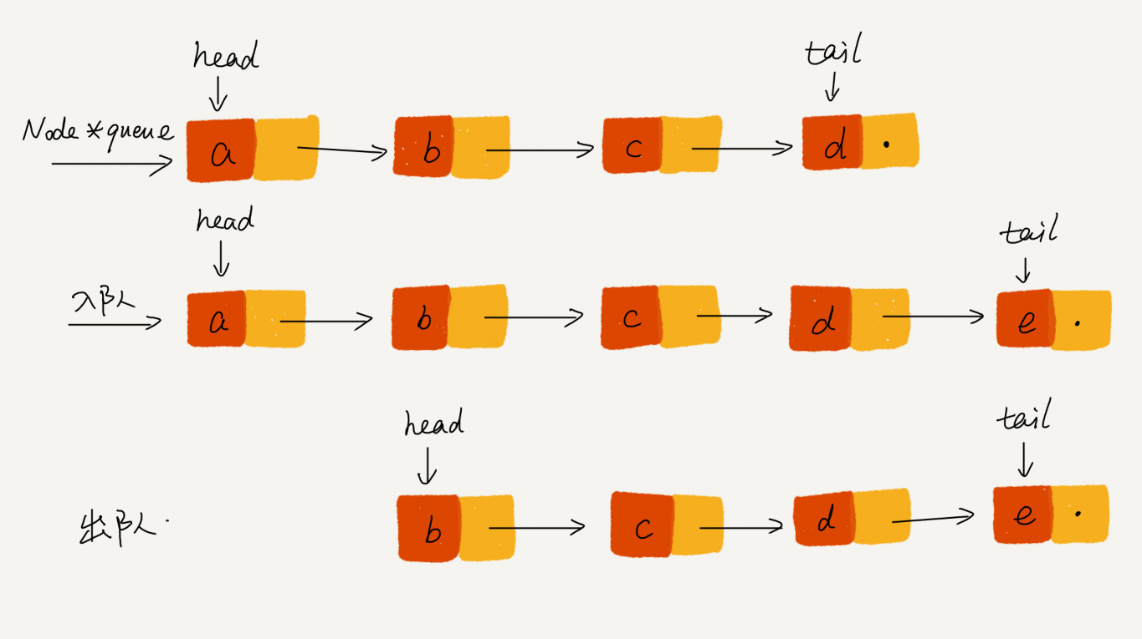### 循环队列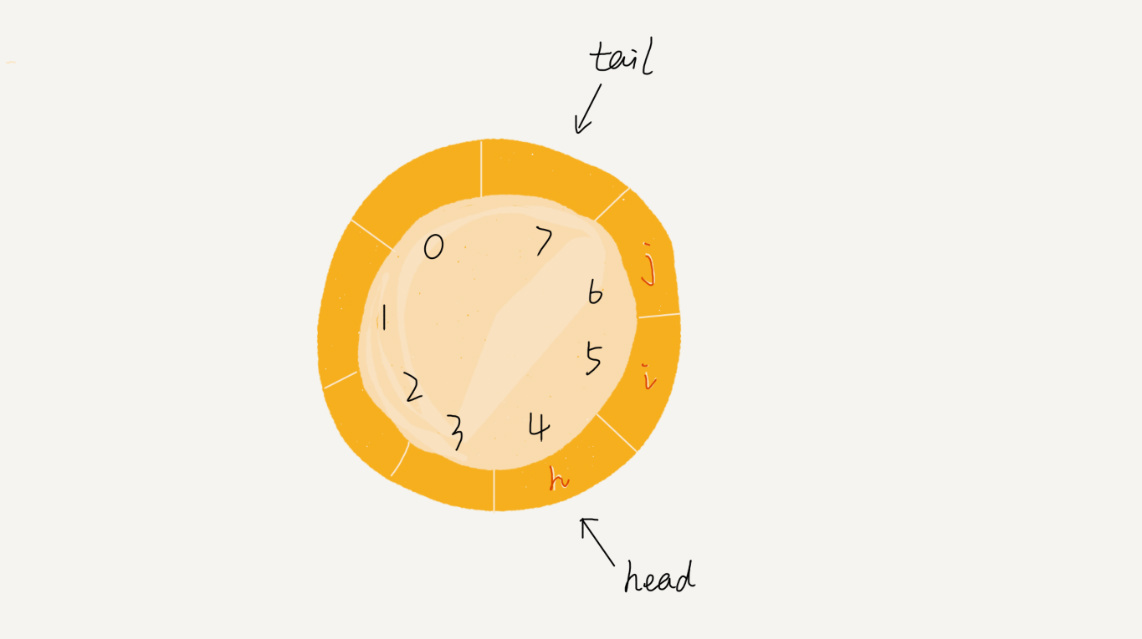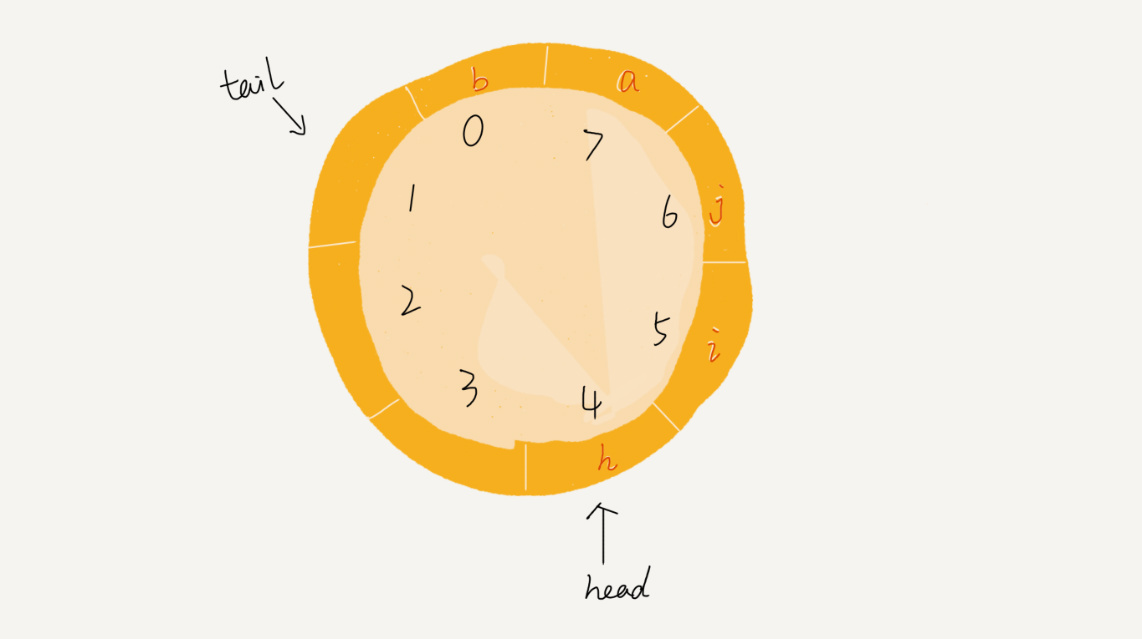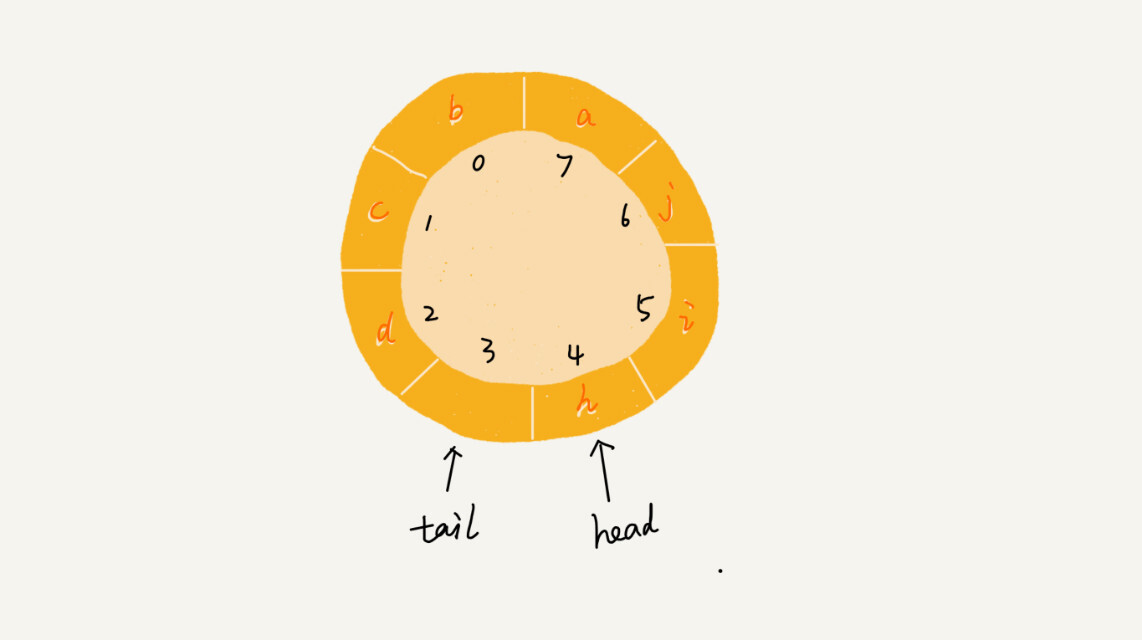``````public class CircularQueue {
// 数组：items，数组大小：n
private String[] items;
private int n = 0;
private int tail = 0;

// 申请一个大小为capacity的数组
public CircularQueue(int capacity) {
items = new String[capacity];
n = capacity;
}

// 入队
public boolean enqueue(String item) {
// 队列满了
if ((tail + 1) % n == head) return false;
items[tail] = item;
tail = (tail + 1) % n;
return true;
}

// 出队
public String dequeue() {
if (head == tail) return null;
return ret;
}
}``````

### 阻塞队列和并发队列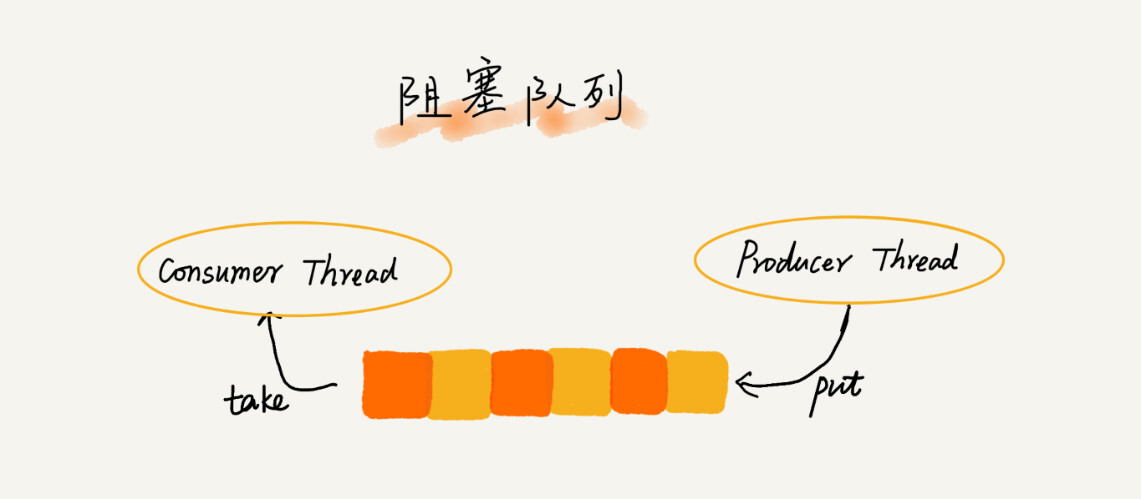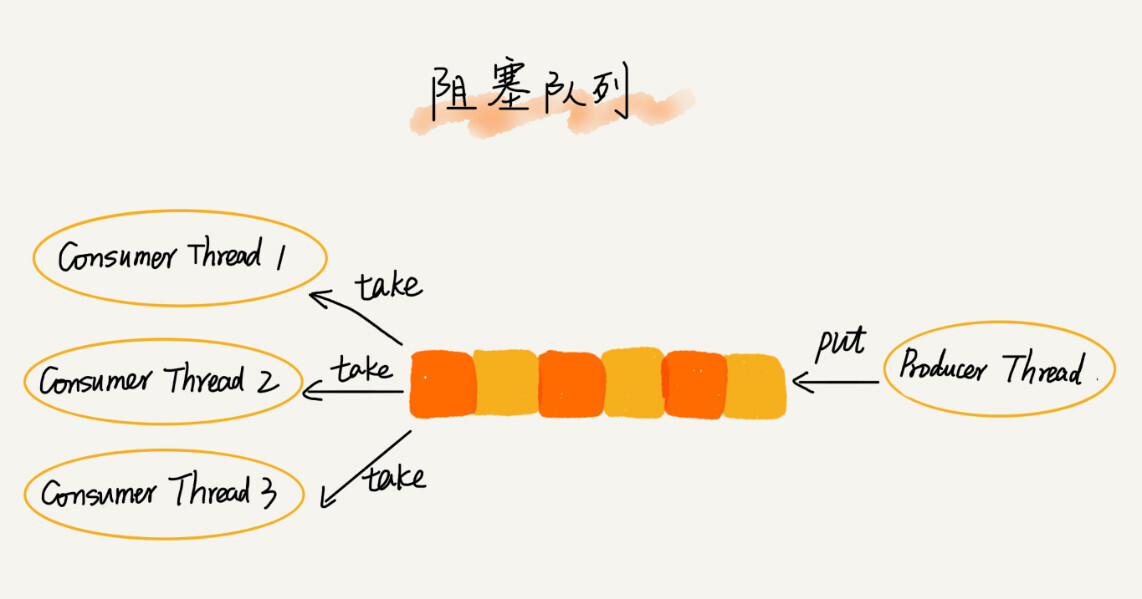## 数据结构与算法五：栈

### 理解“栈”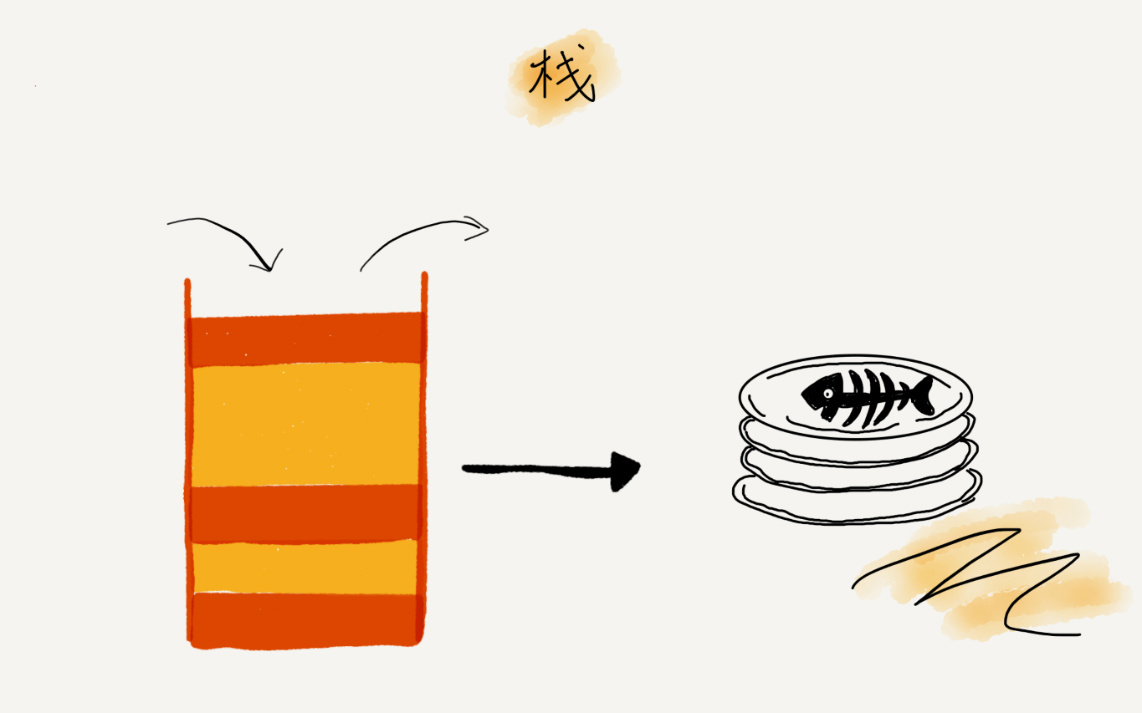### 如何实现一个“栈”？

``````// 基于数组实现的顺序栈
public class ArrayStack {
private String[] items;  // 数组
private int count;       // 栈中元素个数
private int n;           //栈的大小

// 初始化数组，申请一个大小为n的数组空间
public ArrayStack(int n) {
this.items = new String[n];
this.n = n;
this.count = 0;
}

// 入栈操作
public boolean push(String item) {
// 数组空间不够了，直接返回false，入栈失败。
if (count == n) return false;
// 将item放到下标为count的位置，并且count加一
items[count] = item;
++count;
return true;
}

// 出栈操作
public String pop() {
// 栈为空，则直接返回null
if (count == 0) return null;
// 返回下标为count-1的数组元素，并且栈中元素个数count减一
String tmp = items[count-1];
--count;
return tmp;
}
}``````

### 支持动态扩容的顺序栈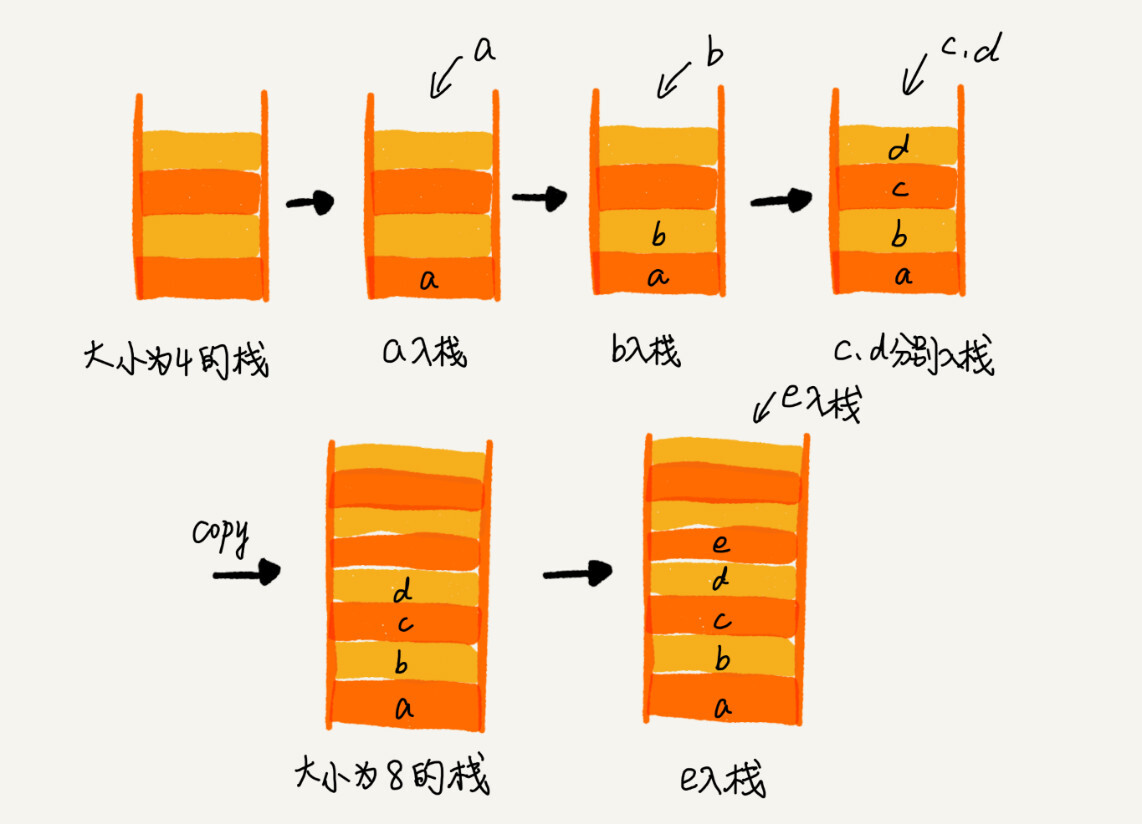• 栈空间不够时，我们重新申请一个是原来大小两倍的数组
• 为了简化分析，假设只有入栈操作没有出栈操作
• 定义不设计内存搬移的入栈操作为 simple-push 操作，时间复杂度为O(1)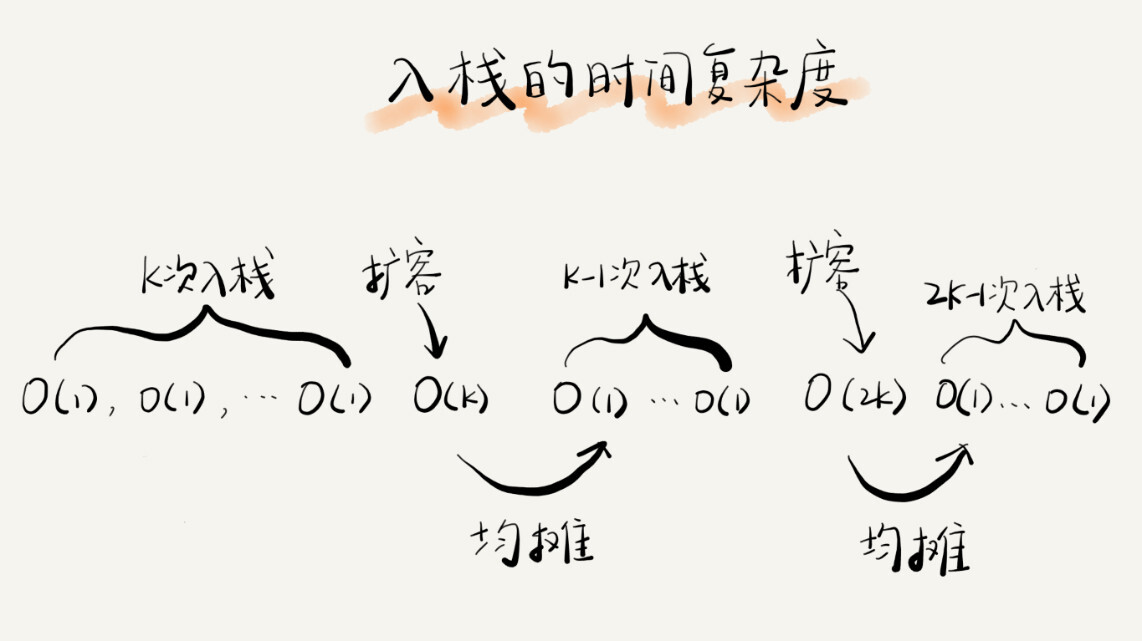### 栈在函数调用中的应用

``````int main() {
int a = 1;
int ret = 0;
int res = 0;
res = a + ret;
printf("%d", res);
reuturn 0;
}

int add(int x, int y) {
int sum = 0;
sum = x + y;
return sum;
}``````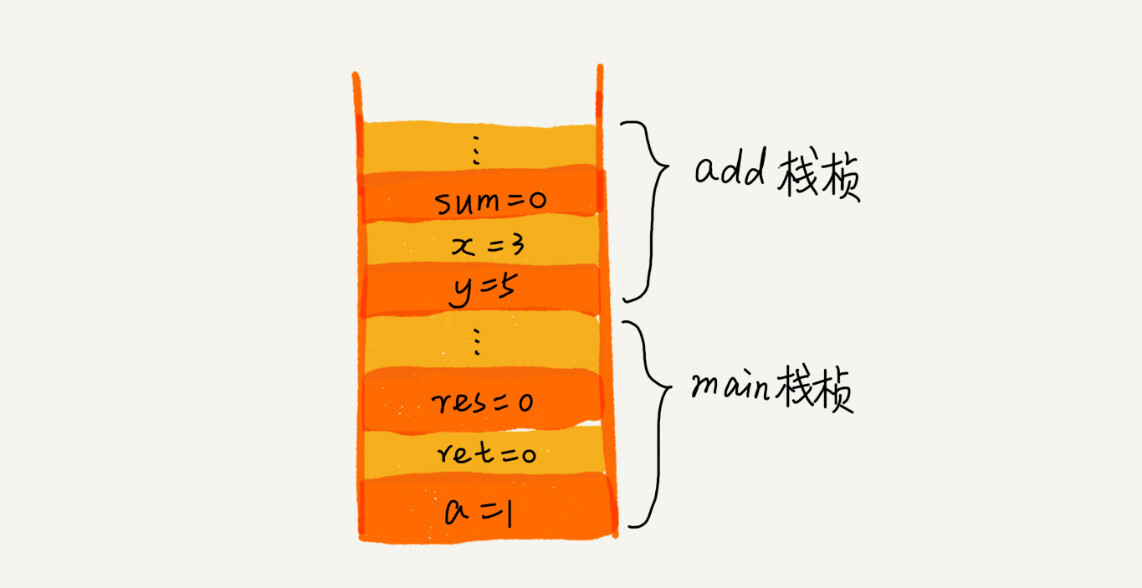### 栈在表达式求值中的应用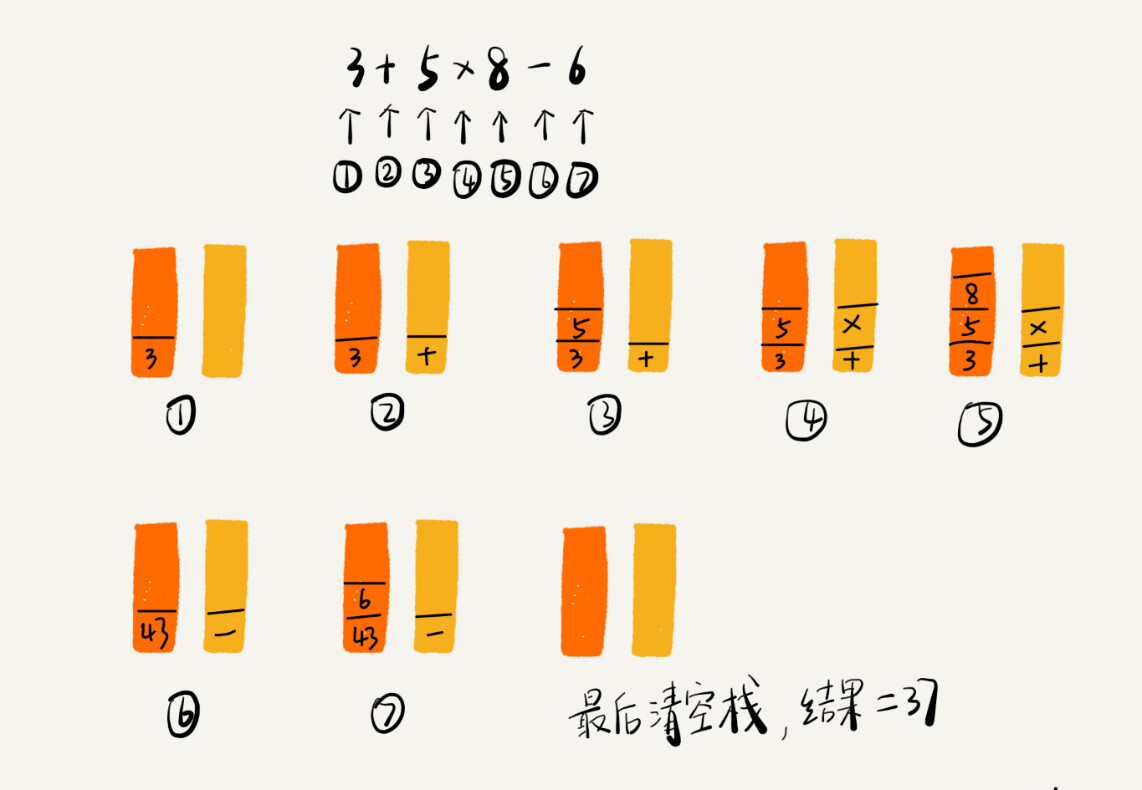### 实现浏览器的前进、后退功能## 数据结构与算法四：链表（下）

### 二：警惕指针丢失和内存泄露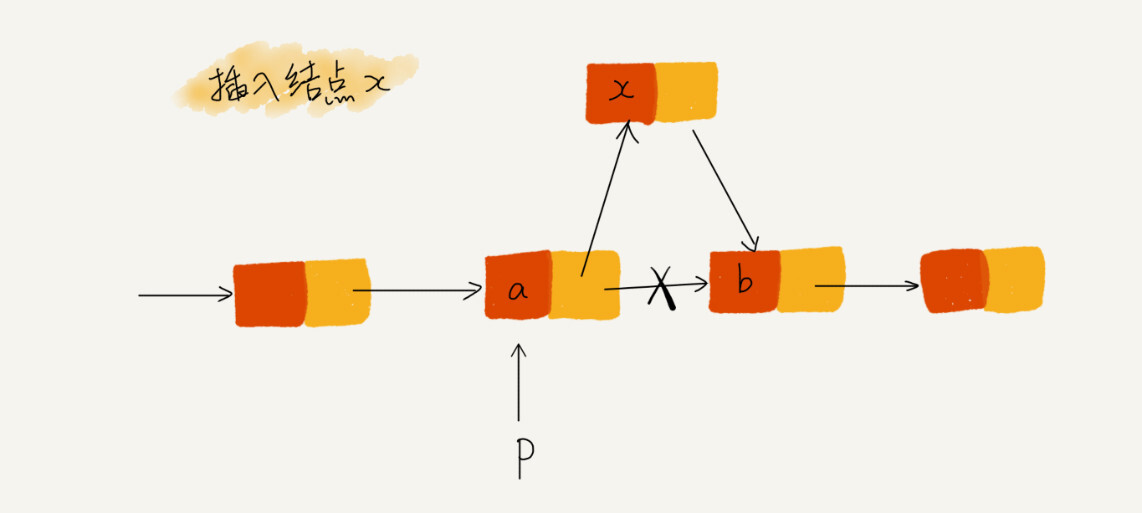``````p->next = x;  // 将p的next指针指向x结点；
x->next = p->next;  // 将x的结点的next指针指向b结点；``````

p->next指针在完成第一步操作之后，已经不再指向结点b了，而是指向了结点x。第二行代码相当于将x复制给x->next，自己指向自己。因此，整个链表也就断成了两半，从结点b往后的所有结点都无法访问到了。

### 三：利用哨兵简化实现难度

``````new_node->next = p->next;
p->next = new_node;``````

``````if (head == null) {
}``````

``p->next = p->next->next;``

``````if (head->next == null) {
}``````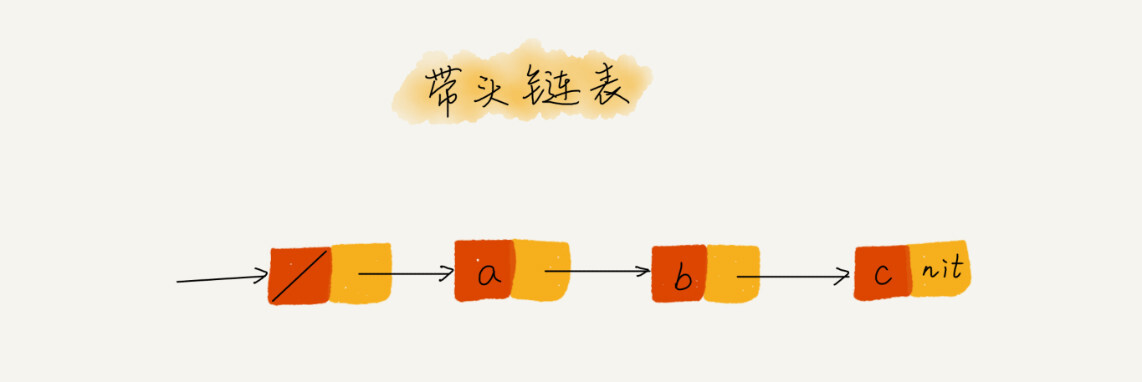``````// 在数组a中，查找key，返回key所在的位置
// 其中，n表示数组a的长度
int find(char* a, int n, char key) {
// 边界条件处理，如果a为空，或者n<=0，说明数组中没有数据，就不用while循环比较了
if(a == null || n <= 0) {
return -1;
}

int i = 0;
// 这里有两个比较操作：i<n和a[i]==key.
while (i < n) {
if (a[i] == key) {
return i;
}
++i;
}

return -1;
}``````

``````// 在数组a中，查找key，返回key所在的位置
// 其中，n表示数组a的长度
// 我举2个例子，你可以拿例子走一下代码
// a = {4, 2, 3, 5, 9, 6}  n=6 key = 7
// a = {4, 2, 3, 5, 9, 6}  n=6 key = 6
int find(char* a, int n, char key) {
if(a == null || n <= 0) {
return -1;
}

// 这里因为要将a[n-1]的值替换成key，所以要特殊处理这个值
if (a[n-1] == key) {
return n-1;
}

// 把a[n-1]的值临时保存在变量tmp中，以便之后恢复。tmp=6。
// 之所以这样做的目的是：希望find()代码不要改变a数组中的内容
char tmp = a[n-1];
// 把key的值放到a[n-1]中，此时a = {4, 2, 3, 5, 9, 7}
a[n-1] = key;

int i = 0;
// while 循环比起代码一，少了i<n这个比较操作
while (a[i] != key) {
++i;
}

// 恢复a[n-1]原来的值,此时a= {4, 2, 3, 5, 9, 6}
a[n-1] = tmp;

if (i == n-1) {
// 如果i == n-1说明，在0...n-2之间都没有key，所以返回-1
return -1;
} else {
// 否则，返回i，就是等于key值的元素的下标
return i;
}
}``````

### 四：重点留意边界条件处理

• 如果链表为空时，代码是否能正常工作？
• 如果链表只包含一个结点时，代码是否能正常工作？
• 如果链表只包含两个结点时，代码是否能正常工作？
• 代码逻辑在处理头结点和尾结点的时候，代码是否能正常工作？

### 五：举例画图，辅助思考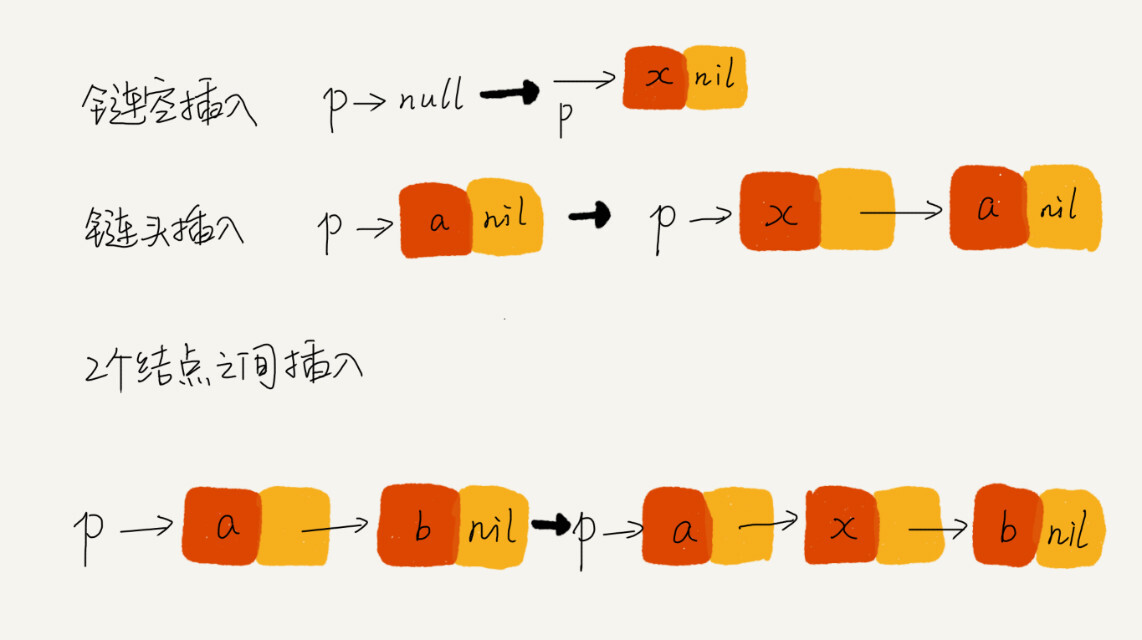• 单链表反转
• 链表中环的检测
• 两个有序的链表合并
• 删除链表倒数第n个结点
• 求链表的中间结点

## 数据结构与算法四：链表（上）

### 链表结构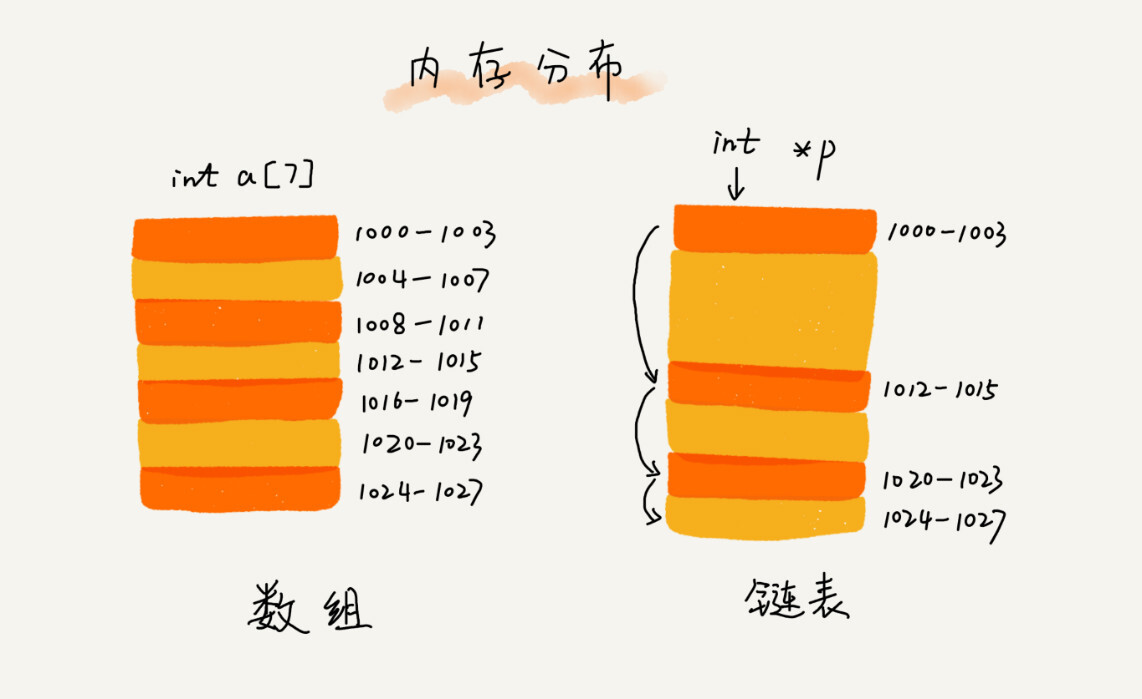### 单链表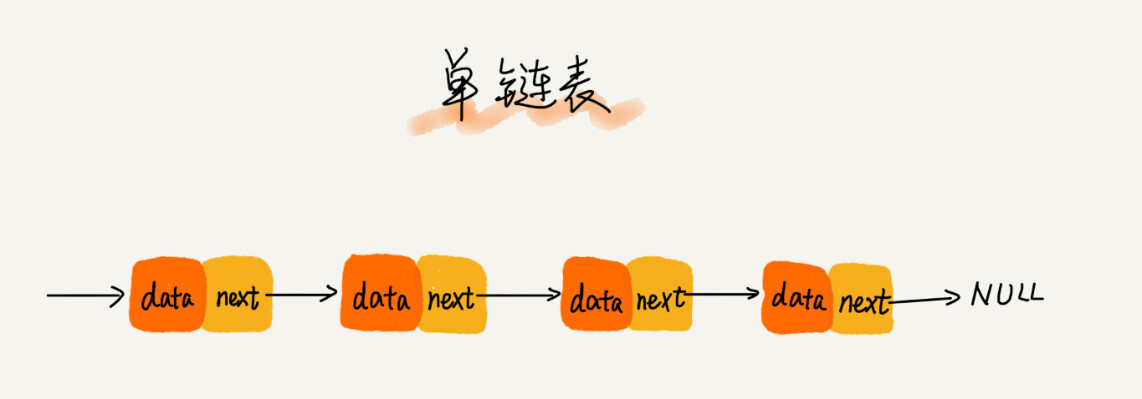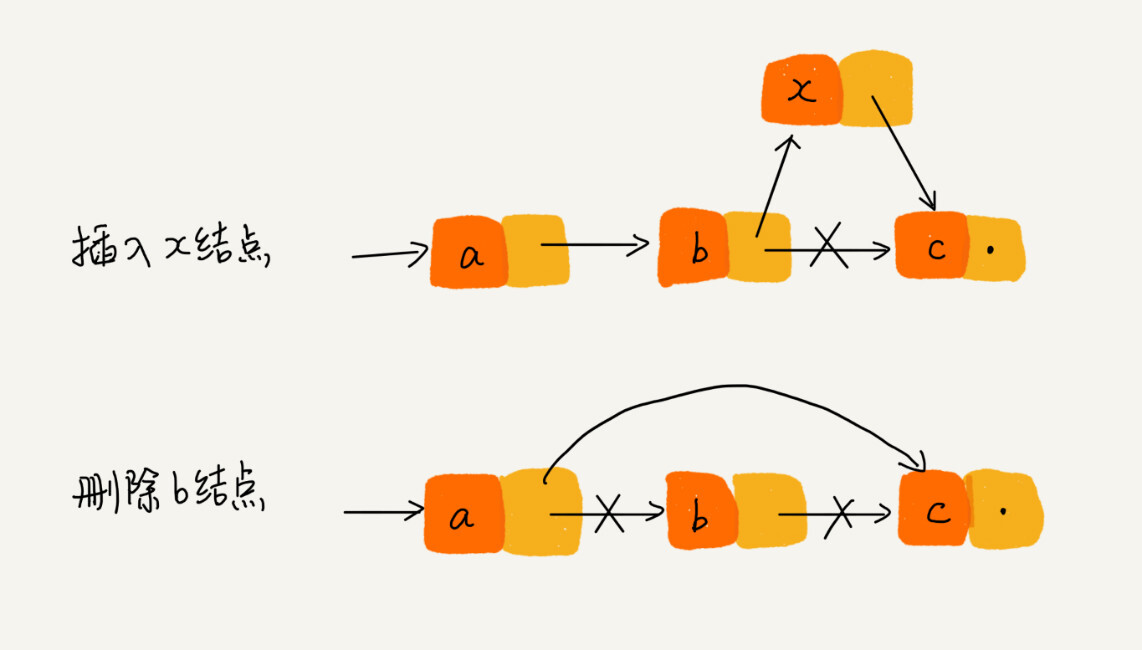### 循环链表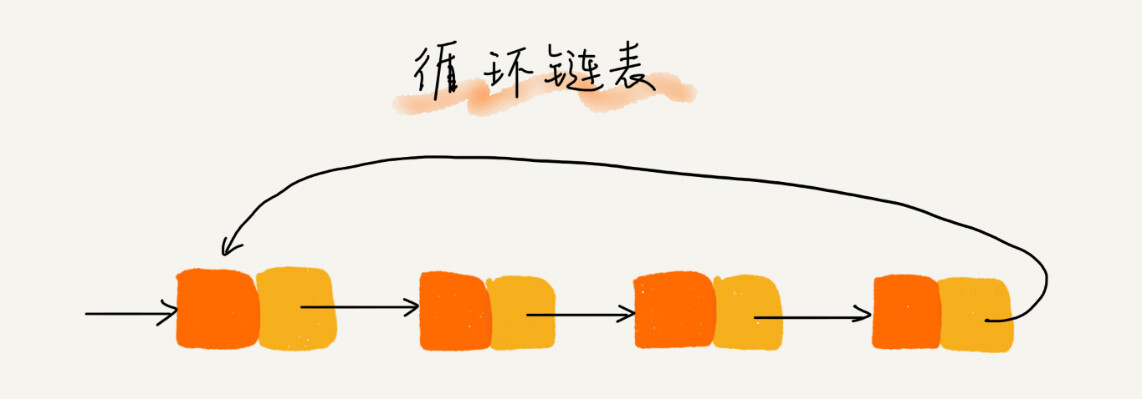### 双向链表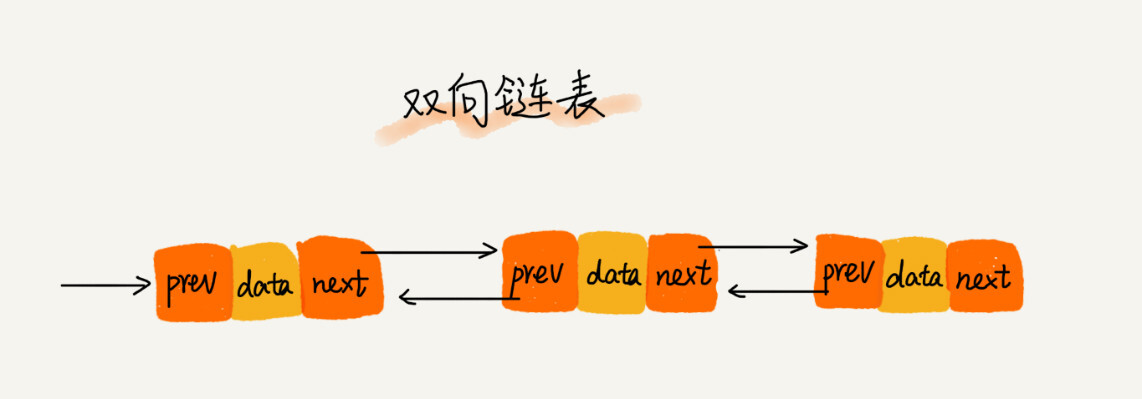### 单链表和双向链表优势

1. 删除节点中“值等于某个给定值”的结点
2. 删除给定指针指向的结点。

### 双向循环链表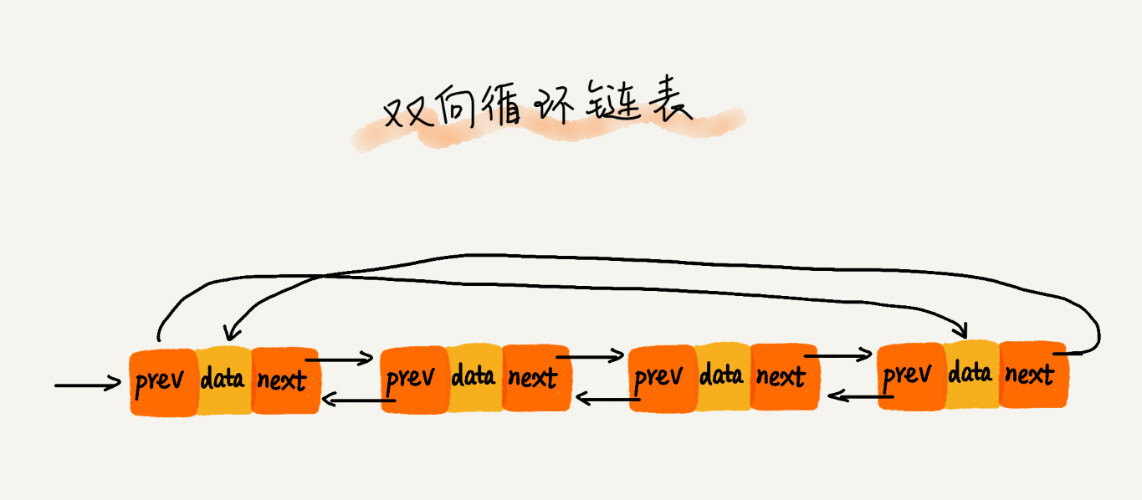### 链表和数组性能比较### LRU缓存淘汰算法

1. 如果此数据之前已经被缓存在链表中了，我们遍历得到这个数据对应的结点，并将其从原来的位置删除，再插入到链表的头部。
2. 如果此数据没有在缓存链表中，又可以分为两种情况：
1. 如果此时缓存未满，则将此结点直接插入到链表的头部；
2. 如果此时缓存已满，则链表尾结点删除，将新的数据插入链表的头部。

## 数据结构与算法三：数组

### 如何实现随机访问？

1. 线性表，数据排成像一条线一样的结构。每个线性表上的数据最多只有前后两个方向。除了数组，链表、队列、栈等也是线性表结构。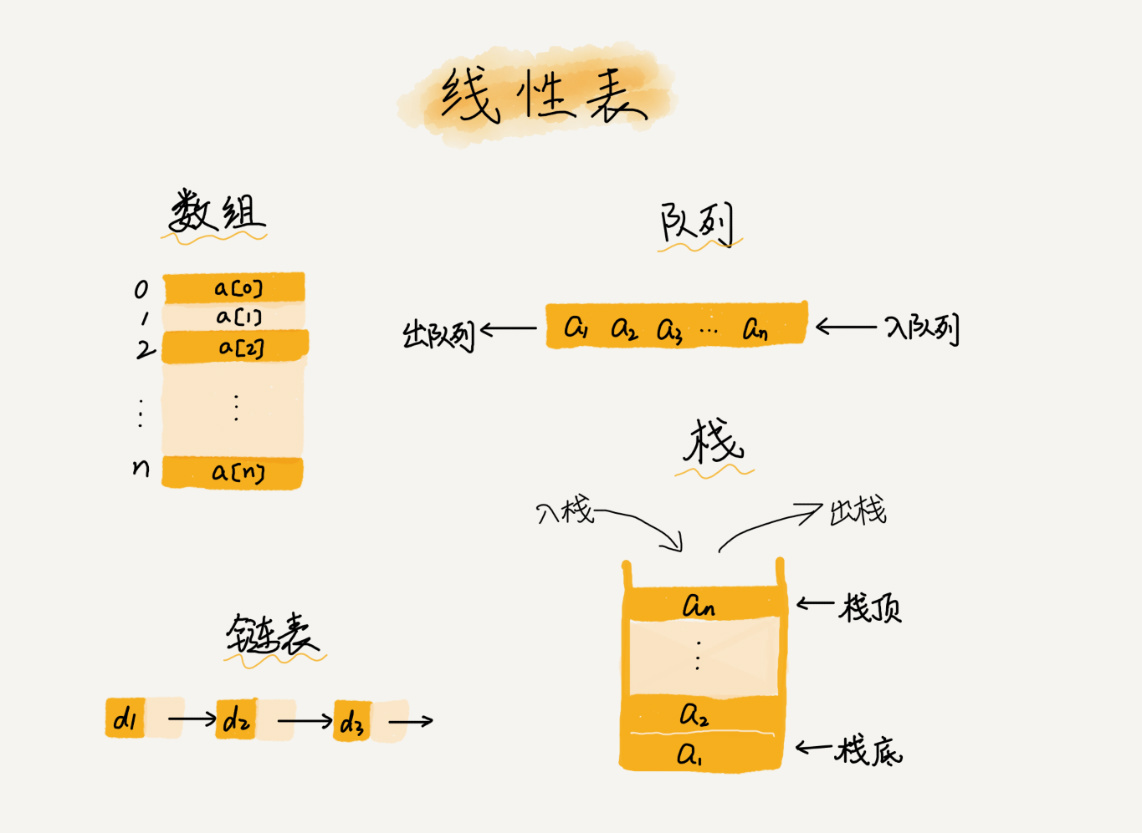而与它相对立的概念是非线性表，比如二叉树、堆、图等。之所以叫非线性表，是因为在非线性表中，数据之间并不是简单的前后关系。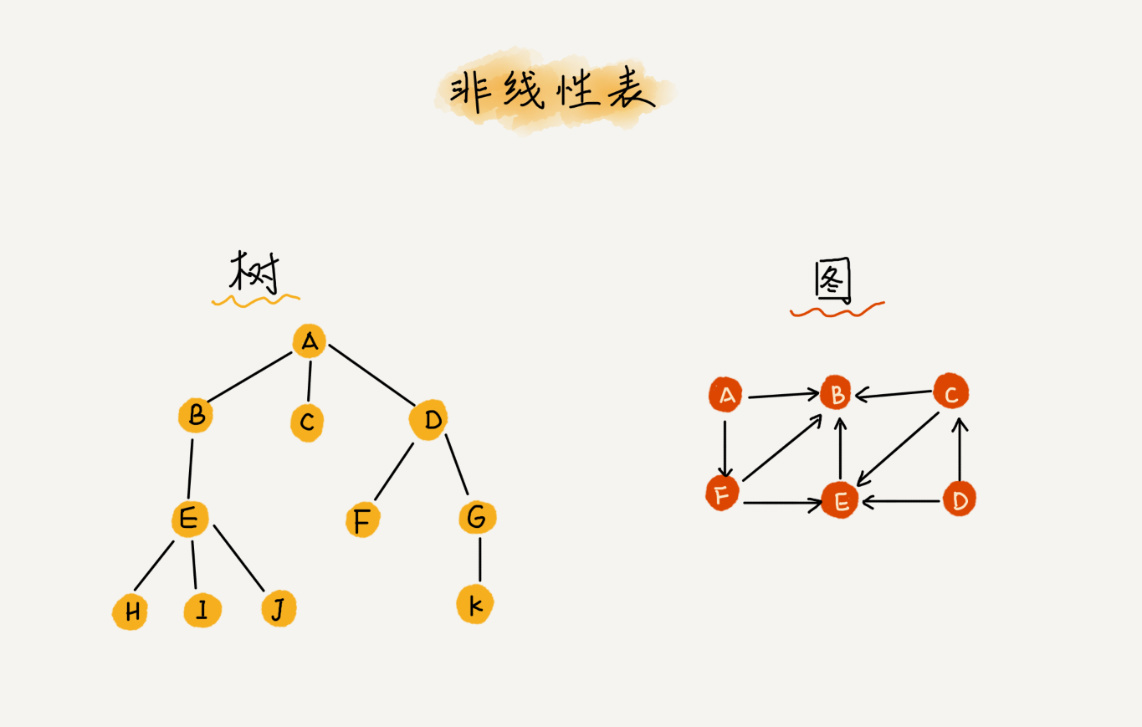2. 连续的内存空间和相同类型的数据。因为这两个限制，数组有了一个堪称“杀手锏”的特性：随机访问。但这两个限制也让数组的很多操作变得低效，比如在数组中删除、插入一个数据，为了保证连续性，就需要做大量的数据搬移工作。

那么数组是如何实现下标随机访问数组元素的呢？拿一个长度为10的int类型的数组 int[] a = new int 来举例。在下图中，计算机给数组 a 分配了一块连续的内存空间 1000 ~ 1039 ，其中，内存块的首地址为base_address = 1000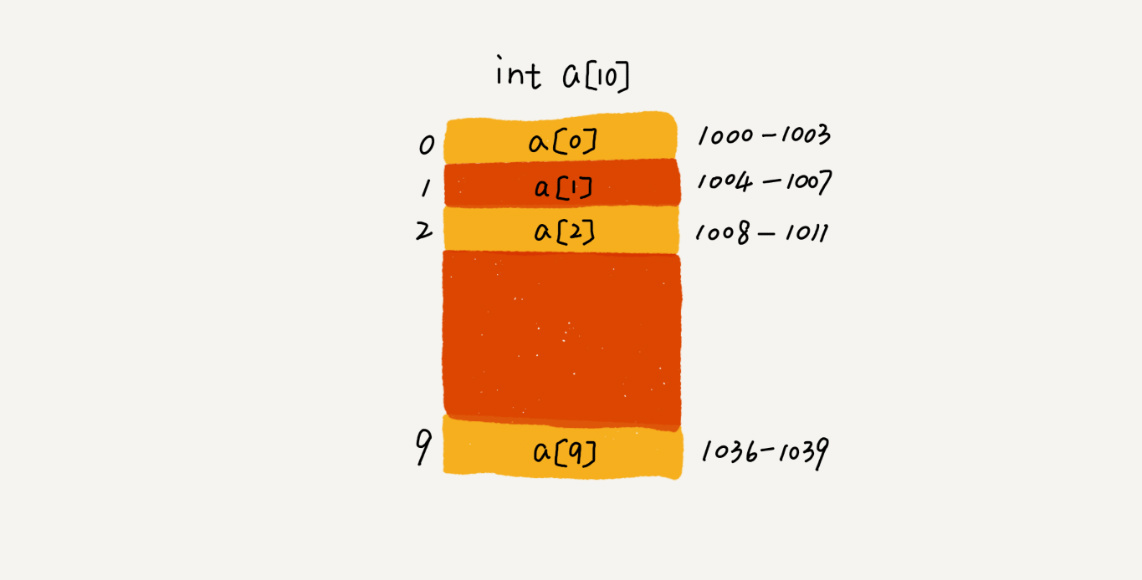我们知道，计算机会给每个内存单元分配一个地址，计算机通过地址来访问内存中的数据。当计算机需要随机访问数组中的某个元素时，它会通过下面的寻址公式，计算出该元素存储的内存地址

``a[i]_address = base_address + i * data_type_size``

其中的 data_type_size 表示数组中每个元素的大小。我们举的这个例子中，数组存储的是 int 类型数据，所以 data_type_size 就为4个字节。

3. 低效的“插入”和“删除”

数组的插入操作，假设数组的长度为n，现在，我们要需要将一个数据插入到数组中的第k个位置。为了把第k个位置腾出来，给新来的数据，我们需要将k~n这部分的元素都顺序的往后挪一位。

如果在数组的末尾插入数据，那就不需要移动数据了，这时的时间复杂度为O(1)。但如果在开头插入元素，所有的数据都需要往后移动一位，所以最欢的时间复杂度为O(n)。因此我们在每个位置插入元素的概率是一样的，所以平均时间复杂度为(1+2+...n)/n=O(n)。

如果数组的数据是有序的，我们在某个位置插入元素的时候就需要用到刚才的方法。但是，如果数组中的数据是没有规律的，数组这是被当成一个存储数据的集合。这种情况下，如果要将某个数据插入到第k个位置，为了避免大规模的数据搬移，我们还有一个简单的办法，将第k位的数据搬移到数组元素的最后，把新的元素直接放入到第k个位置。

为了更好的理解，我们假设数组 a 中存储了5个元素：a，b，c，d，e。我们现在需要将元素x插入到第三个位置。那么我们只需要将c放入到 a ，将 a 赋值为 x 即可。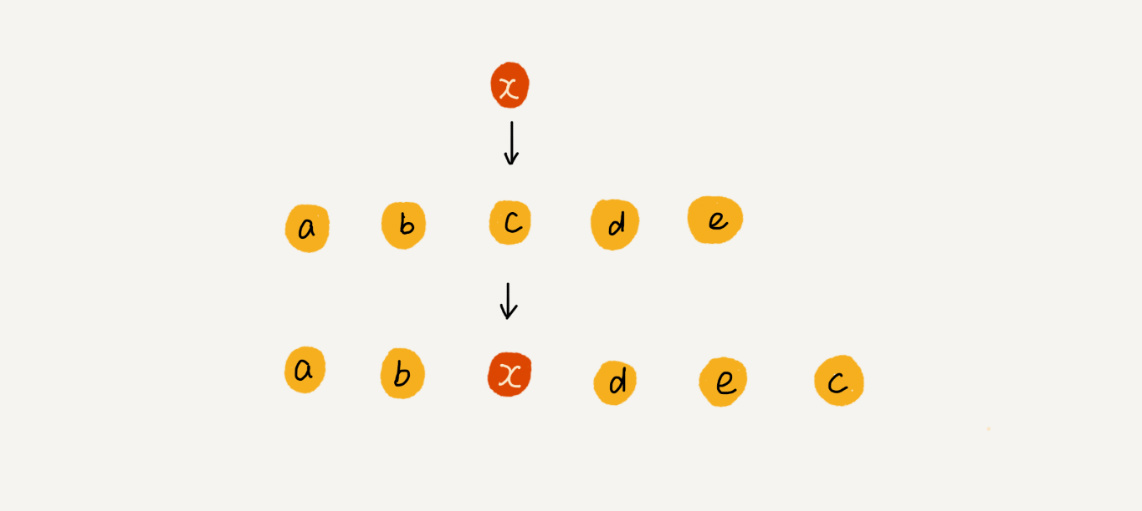删除跟插入类似，我们要删除第k位的元素，为了内存的连续性，我们需要搬移数据，不让内存中间出现空洞不连续。删除末尾的数据，最好时间复杂度为O(1)。删除开头数据，最坏时间复杂度为O(n)，平均时间复杂度也是O(n)。

实际上，在某些特殊场景下，我们不一定非得追求数组中数据的连续性。

例，数组 a 中存储了8个元素：a，b，c，d，e，f，g，h。现在，我们要一词删除a，b，c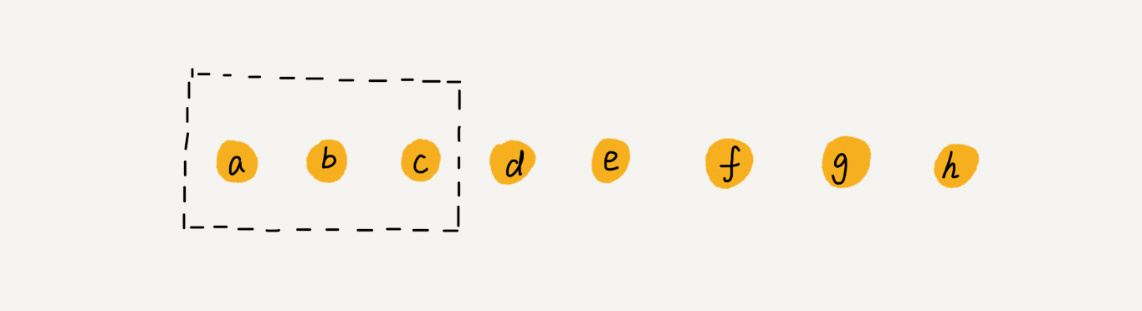为了避免d，e，f，g，h这个几个数据被搬移三次，我们可以先记录下已经删除的数据。每次的删除操作并不是真正的搬移工作，只是记录数据已经被删除。当数组没有更多空间存储数据时，我们再触发执行一次真正的删除操作，这样就大大减少了删除操作导致的数据搬移。这也是JVM标记清楚垃圾回收算法的核心思想。

### 警惕数组的访问越界问题

``````int main(int argc, char* argv[]){
int i = 0;
int arr = {0};
for(; i<=3; i++){
arr[i] = 0;
printf("hello world\n");
}
return 0;
}``````

### 容器能否完全代替数组？

``````1. Java ArrayList无法存储基本类型，比如int、long，需要封装为Integer、Long类，而 Autoboxing、Unboxing 则有一定的性能消耗，所以如果特别关注性能，或者希望使用基本类型，就可以选用数组。
2. 如果事先知道数据大小，并且对数据的操作非常简单，且用不到ArrayList提供的大部分方法，也可以直接使用数组。
3. 当要表示多维数组时，用数组往往会更加直观。比如Object[][] array；而用容器的话则需要这样定义：ArrayList<ArrayList<object>> array。``````

### 为什么数组从0开始编号

``a[k]_address = base_address + k * type_size``

``a[k]_address = base_address + (k-1)*type_size``

## 数据结构与算法二：复杂度分析（下）

### 最好、最坏情况时间复杂度

``````// n表示数组array的长度
int find(int[] array, int n, int x) {
int i = 0;
int pos = -1;
for (; i < n; ++i) {
if (array[i] == x) pos = i;
}
return pos;
}``````

``````// n表示数组array的长度
int find(int[] array, int n, int x) {
int i = 0;
int pos = -1;
for (; i < n; ++i) {
if (array[i] == x) {
pos = i;
break;
}
}
return pos;
}``````

### 平均时间复杂度### 均摊时间复杂度

`````` // array表示一个长度为n的数组
// 代码中的array.length就等于n
int[] array = new int[n];
int count = 0;

void insert(int val) {
if (count == array.length) {
int sum = 0;
for (int i = 0; i < array.length; ++i) {
sum = sum + array[i];
}
array = sum;
count = 1;
}

array[count] = val;
++count;
}``````## 数据结构与算法二：复杂度分析（上）

### 大O复杂度表示法(时间复杂度)

1. 只关注循环执行次数最多的一段代码

我们在分析一个算法、一段代码的时间复杂度的时候，也只关注循环次数最多的那段代码就可以了

``````int cal(int n) {
int sum = 0;
int i = 1;
for (; i <= n; ++i) {
sum = sum + i;
}
return sum;
}``````

当n很大时，可以想象成10000，1000000。而代码中的低阶、常量、系数三个部分并不左右增长趋势，所以可以忽略。我们只需要记录一个最大量级就可以了。

第二三行都是常量级的执行时间，与n的大小无关，所以对于时间复杂度没有影响。执行时间最多的是第四五行代码，都被执行了n次，所以时间复杂度为O(n)

2. 加法法则，总复杂度等于量级最大的那段代码的复杂度

``````int cal(int n) {
int sum_1 = 0;
int p = 1;
for (; p < 100; ++p) {
sum_1 = sum_1 + p;
}

int sum_2 = 0;
int q = 1;
for (; q < n; ++q) {
sum_2 = sum_2 + q;
}

int sum_3 = 0;
int i = 1;
int j = 1;
for (; i <= n; ++i) {
j = 1;
for (; j <= n; ++j) {
sum_3 = sum_3 +  i * j;
}
}

return sum_1 + sum_2 + sum_3;
}``````

这段代码分为三个部分，sum_1、sum_2、sum_3，我们可以分析每一部分的时间复杂度，然后把它们放到一块儿，再取一个量级最大的作为整段代码的时间复杂度。

第一部分执行了100次，所以是一个常量的执行时间，与n的规模无关，所以这里的时间复杂度记为O(1)

第二部分时间复杂度 O(n)，第三部分时间复杂度 O(n²)。综合三段代码，取其中最大的量级，所以这段代码的时间复杂度为O(n²)

3. 乘法法则：嵌套代码的复杂度等于嵌套内外代码复杂度的乘积

``````int cal(int n) {
int ret = 0;
int i = 1;
for (; i < n; ++i) {
ret = ret + f(i);
}
}

int f(int n) {
int sum = 0;
int i = 1;
for (; i < n; ++i) {
sum = sum + i;
}
return sum;
}``````

单看cal函数，时间复杂度为O(n)。但f函数不是一个简单的操作，f函数的时间复杂度可以看出为O(n)，所以，整个cal函数内的时间复杂度为两个复杂度的成绩O(n²)

### 几种常见的时间复杂度分析1. O(1)
首先要明确一个概念，O(1)这是常量级时间复杂度的一种表示方式，并不是指只执行了一段代码

``````int i = 8;
int j = 6;
int sum = i + j;``````

一般情况下，只要算法中不存在循环语句、递归语句，即使有成千上万行代码，时间复杂度也是O(1)

2. O(logn)、O(nlogn)

``````i=1;
while (i <= n)  {
i = i * 2;
}``````

从代码中看出，i从1开始取，每循环一次乘以2，由此得出：所以，我们只需要知道x是多少，就能知道这段代码的执行次数了。通过2^x=n可以得出，x=log(2)n，所以这段代码的时间复杂度为log(2)n。

我们知道，对数之间是可以互相转换的 log(3)n = log(3)2 log(2)n，所以O(log(3)n) = O(log(3)2 log(2)n)，其中Log(3)2是常量，可以忽略系数，所以log(3)n = log(2)n。

在对数的时间复杂度的表示法里，我们忽略对数的底，统一表示为O(logn)。所以这段代码的最终时间复杂度为O(logn)

利用乘法法则，我们可以很轻易的了解到O(nlogn)，即如果一段时间复杂度为O(logn)的代码执行了n次，时间复杂度就为O(nlogn)了。O(nlogn)是一种非常常见的时间复杂度，比如，归并排序、快速排序。

3. O(m+n)、O(m*n)

``````int cal(int m, int n) {
int sum_1 = 0;
int i = 1;
for (; i < m; ++i) {
sum_1 = sum_1 + i;
}

int sum_2 = 0;
int j = 1;
for (; j < n; ++j) {
sum_2 = sum_2 + j;
}

return sum_1 + sum_2;
}``````

这段代码分为两个部分，第一部分的时间复杂度为O(m)，第二部分的时间复杂度为O(n)。但我们无法评估m和n谁的量级大，所以我们在表示时间复杂度的时候，就不能简单的利用加法法则省略掉其中一个。所以，这段代码的时间复杂度为O(m+n)。

这种情况的加法法则就不正确了，时间复杂度改为了m+n，但乘法法则依然有效。

### 空间复杂度分析

``````void print(int n) {
int i = 0;
int[] a = new int[n];
for (i; i <n; ++i) {
a[i] = i * i;
}

for (i = n-1; i >= 0; --i) {
print out a[i]
}
}``````

## 数据结构与算法一：初识

1. 广义概念

1. 狭义概念

### 学习重点

1. 复杂度分析

1. 正文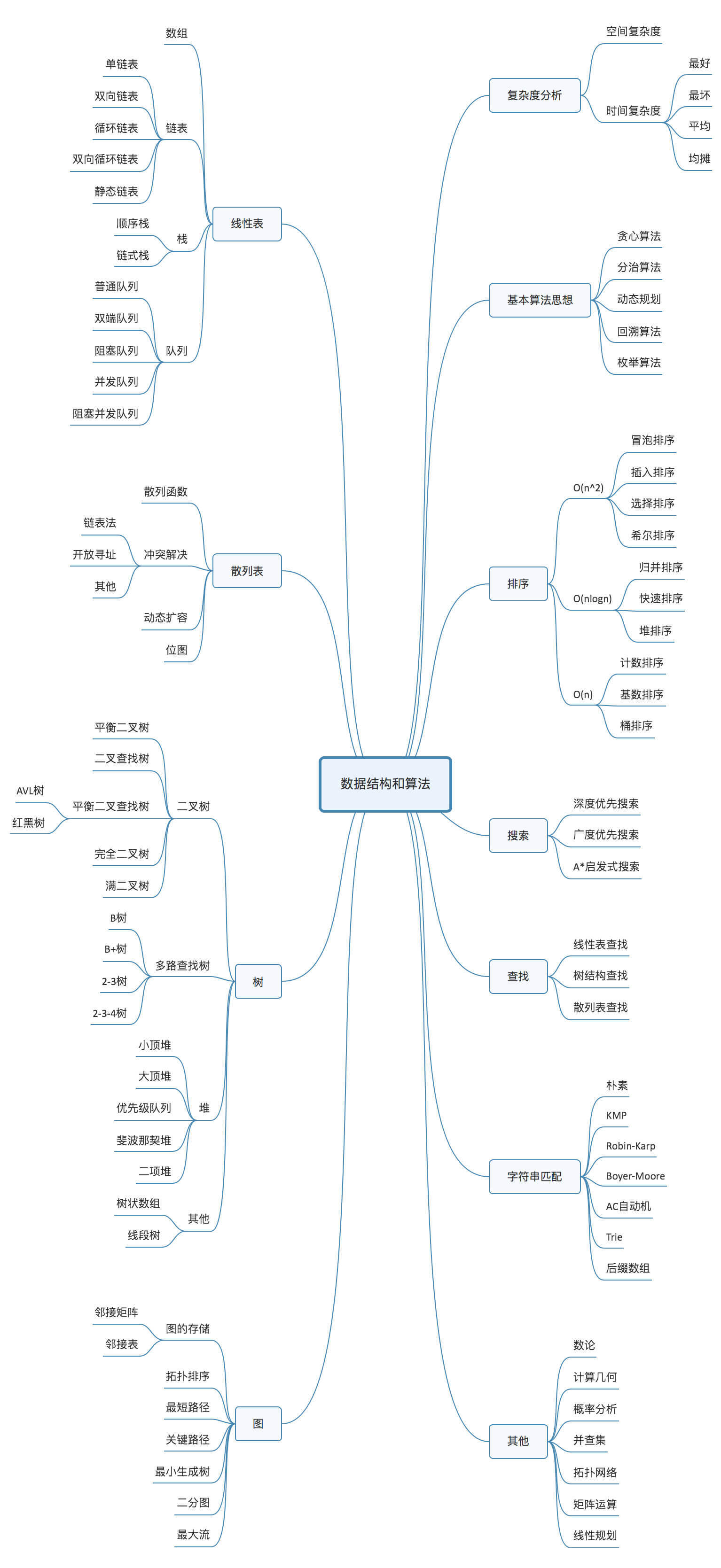• 10个数据结构：数组、链表、栈、队列、散列表、二叉树、堆、跳表、图、Trie树
• 10个算法：递归、排序、二分查找、搜索、哈希算法、贪心算法、分治算法、回溯算法、动态规划、字符串匹配算法

## RabbitMQ的实现

### 生产者：

```\$config = [
'host' => '127.0.0.1',
'vhost' => 'rabbitmqhost',
'port' => 5672,
];

\$exchangeName = 'kd_sms_send_ex'; //交换机名
\$queueName = 'kd_sms_send_q'; //队列名称
\$routingKey = 'sms_send'; //路由关键字(也可以省略)

// 建立生产者与mq之间的连接
\$conn = new AMQPStreamConnection(
);
// 在已连接基础上建立生产者与mq之间的通道
\$ch = \$conn->channel();

// 声明初始化交换机
\$ch->exchange_declare(\$exchangeName, 'direct', false, true, false);
// 声明初始化一条队列
\$ch->queue_declare(\$queueName, false, true, false, false);
// 将队列与某个交换机进行绑定，并使用路由关键字
\$ch->queue_bind(\$queueName, \$exchangeName, \$routingKey);

\$msgBody = json_encode(["name" => "iGoo", "age" => 22]);
// 生成消息
\$msg = new AMQPMessage(\$msgBody, ['content_type' => 'text/plain', 'delivery_mode' => 2]);
// 推送消息到某个交换机
\$ch->basic_publish(\$msg, \$exchangeName, \$routingKey);```

### 消费者：

```\$config = [
'host' => '127.0.0.1',
'vhost' => 'rabbitmqhost',
'port' => 5672,
];

// 交换机名
\$exchangeName = 'kd_sms_send_ex';
// 队列名称
\$queueName = 'kd_sms_send_q';
// 路由关键字(也可以省略)
\$routingKey = 'sms_send';

// 建立生产者与mq之间的连接
\$conn = new AMQPStreamConnection(
);
// 在已连接基础上建立生产者与mq之间的通道
\$ch = \$conn->channel();

// 声明初始化一条队列
\$ch->queue_declare(\$queueName, false, true, false, false);

// 在接收消息的时候调用回调函数
\$ch->basic_consume(\$queueName, '', false, true, false, false, function (\$msg){
vdump(" [x] Received ", \$msg->body, "\n");
});

// 堵塞
while(count(\$ch->callbacks)) {
\$ch->wait();
}```

## PHPstorm常用快捷键

 CTRL+N 查找类 CTRL+SHIFT+N 全局搜索文件 ,优先文件名匹配的文件 CTRL+SHIFT+ALT+N 查找php类名/变量名 ,js方法名/变量名, css 选择器 CIRL+B 找变量的来源，跳到变量申明处 CTRL+G 定位行，跳转行 CTRL+J 自动代码提示，自动补全 ALT+回车 导入包,自动修正 CTRL+ALT+L 格式化代码 CTRL+ALT+I 自动缩进 CTRL+ALT+SPACE 类名或接口名提示（与系统冲突） 提示类名关键字 (abstract public ...) CTRL+P 方法参数提示，显示默认参数 CTRL+ALT+O 优化导入的类和包 需要配置 CTRL+W 块状选中代码